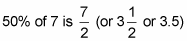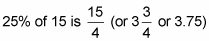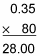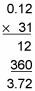##### Basic Math & Pre-Algebra All-in-One For Dummies (+ Chapter Quizzes Online)A lot of percent problems turn out to be easy to solve when you give them a little thought. In many cases, just remember the connection between percents and fractions and you’re halfway home.

## Solve simple percent problems

Some percents are easy to figure. Here are a few.
• Finding 100% of a number: Remember that 100% means the whole thing, so 100% of any number is simply the number itself:

100% of 5 is 5

100% of 91 is 91

100% of 732 is 732

• Finding 50% of a number: Remember that 50% means half, so to find 50% of a number, just divide it by 2:

50% of 20 is 10

50% of 88 is 44• Finding 25% of a number: Remember that 25% equals 1/4, so to find 25% of a number, divide it by 4:

25% of 40 is 10

25% of 88 is 22• Finding 20% of a number: Finding 20% of a number is handy if you like the service you’ve received in a restaurant, because a good tip is 20% of the check. Because 20% equals 1/5, you can find 20% of a number by dividing it by 5. But you can use an easier way:

To find 20% of a number, move the decimal point one place to the left and double the result:

20% of 80 = 8 2 = 16

20% of 300 = 30 2 = 60

20% of 41 = 4.1 2 = 8.2

• Finding 10% of a number: Finding 10% of any number is the same as finding 1/10 of that number. To do this, just move the decimal point one place to the left:

10% of 30 is 3

10% of 41 is 4.1

10% of 7 is 0.7

• Finding 200%, 300%, and so on of a number: Working with percents that are multiples of 100 is easy. Just drop the two 0s and multiply by the number that’s left:

200% of 7 = 2 7 = 14

300% of 10 = 3 10 = 30

1,000% of 45 = 10 45 = 450

## Make tough-looking percent problems easy

Here’s a trick that makes certain tough-looking percent problems so easy that you can do them in your head. Simply move the percent sign from one number to the other and flip the order of the numbers.

Suppose someone wants you to figure out the following:

88% of 50

Finding 88% of anything isn’t an activity that anybody looks forward to. But an easy way of solving the problem is to switch it around:

88% of 50 = 50% of 88

This move is perfectly valid, and it makes the problem a lot easier. As you learned above, 50% of 88 is simply half of 88:

88% of 50 = 50% of 88 = 44

As another example, suppose you want to find

7% of 200

Again, finding 7% is tricky, but finding 200% is simple, so switch the problem around:

7% of 200 = 200% of 7

Above, you learned that to find 200% of any number, you just multiply that number by 2:

7% of 200 = 200% of 7 = 2 7 = 14

## Solve more-difficult percent problems

You can solve a lot of percent problems using the tricks shown above. But what about this problem?

35% of 80 = ?

Ouch — this time, the numbers you’re working with aren’t so friendly. When the numbers in a percent problem become a little more difficult, the tricks no longer work, so you want to know how to solve all percent problems.

Here’s how to find any percent of any number:

1. Change the word of to a multiplication sign and the percent to a decimal.

Changing the word of to a multiplication sign is a simple example of turning words into numbers. This change turns something unfamiliar into a form that you know how to work with.

So, to find 35% of 80, you would rewrite it as:

35% of 80 = 0.35 80

2. Solve the problem using decimal multiplication.

Here’s what the example looks like:So 35% of 80 is 28.

As another example, suppose you want to find 12% of 31. Again, start by changing the percent to a decimal and the word of to a multiplication sign:

12% of 31 = 0.12 31

Now you can solve the problem with decimal multiplication:So 12% of 31 is 3.72.